## Two balls are thrown against a wall. Ball 1 has a much higher speed than ball 2. Explain how the energy of the two balls is different

Question

Two balls are thrown against a wall. Ball 1 has a much higher speed than ball 2.
Explain how the energy of the two balls is different.

in progress 0
6 months 2021-07-15T02:42:10+00:00 1 Answers 51 views 0

1. Let both the balls have the same mass equals to m.

Let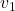and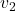be the speed of the ball1 and the ball2 respectively, such that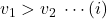Assuming that both the balls are at the same level with respect to the ground, so let h be the height from the ground.

The total energy of ball1= Kinetic energy of ball1 + Potential energy of ball1. The Kinetic energy of any object moving with speed,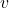, is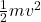and the potential energy is due to the change in height is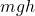[where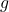is the acceleration due to gravity]

So, the total energy of ball1,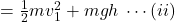and the total energy of ball1,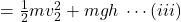.

Here, the potential energy for both the balls are the same, but the kinetic energy of the ball1 is higher the ball2 as the ball1 have the higher speed, refer equation (i)

So,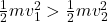Now, from equations (ii) and (iii)

The total energy of ball1 hi higher than the total energy of ball2.